# Discussion of the Pi=4 problem from the New York Times

This was written by Zandra Vinegar on March 18, 2016 at the New York Times article here.

Part 1: Pointwise Convergence

The key to the general method for distinguishing between valid and invalid convergence proofs that $\pi = x$ is going to be a concept known as "uniform convergence."

Normal convergence or, "pointwise convergence" is when each point in the deforming figure/function gets closer and closer to a target value/position each stage. Or, more formally:

Let $S$ be a subset of the real numbers, $R,$ and let $F_n(x)$ be an infinite sequence of functions $(n = 1, 2, 3 \cdots)$ defined on $S.$ We say that $F_n$ converges pointwise on $S$ to the function $G(x)$ if the limit as $n$ approaches infinity of $F_n(x)$ exists and equals $G(x)$ for each point $x$ in $S.$

Most intuition stops here (at least, mine does - and it tells me that if the above is true, then in all practical ways, the $F_n(x)$series converges to become $G(x)$. However, that's just not true. You see, there's a tricky way to make a series of functions so that, although every point approaches the right limit, there are a few singularities near to which points approach the limit only after many, many stages. And the effect we see is a crinkle in our function that gets pushed to a tinier and tinier range, but which never disappears entirely.

Part 2: Uniform Convergence

Universal convergence forces out this crinkle in a beautifully simple way: we simply explain that what we really care about is the WORST behaved point at each stage. And if there isn't a "worst" point, we demand controlling the boundary or limit of the worst cases. And we require that this boundary containing all of the horribleness/error converge to nothing, in limit as the number of stages approaches infinity.

Formally:

Let $S$ be a subset of the real numbers, $R,$ and let $F_n(x)$ be a sequence of functions defined on $S.$ We say that $F_n(x)$ converges uniformly on $S$ to the function $G(x)$ if, given any $\epsilon > 0,$ there exists a natural number $N$ such that $|F_n(x) - G(x)| < \epsilon$ for every $n > N$ and for every $x$ in $S.$

In other words, I prove that a function converges uniformly if I can show how, for any margin of error $(\epsilon)$ that you might give me, I can find a stage at which the ENTIRE function, $F_n(x)$ is within an error bound of epsilon from $G(x)$ at every point $x.$

[ED. NOTE: As you will see later, to approximate the perimeter, we will want uniform convergence of $\frac{dx}{dt}$ and $\frac{dy}{dt}$.

Part 3: Gathering the False Proofs

When I'm staring at a false proof that I'm tempted to believe at some level, I find that it grounds my perspective to suss out exactly what the proof /does/ prove, compared to what it claims.

Here are our false proofs:

1) The Square Noose: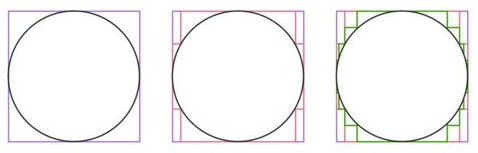1.5) A lower bound follow-up to the square noose: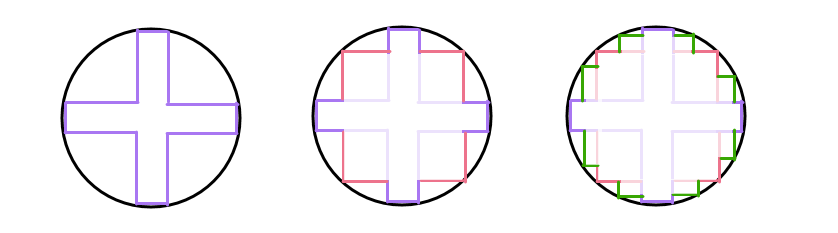2) The Spiral of Doom: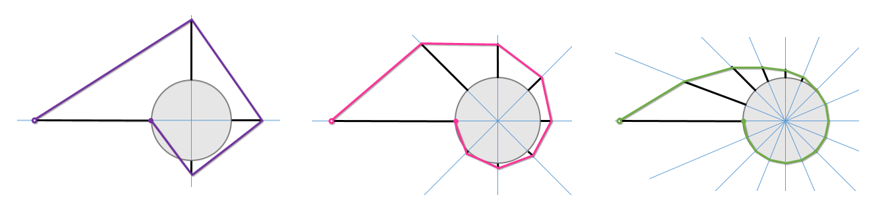== PICKING APART PROOF 1 ==

The common intuition used in all of these proofs is that, by getting closer to the circle at every state, the series of constructed figures is literally /becoming/ the circle.

Sometimes, such as in the series .5 + .25 + .125 +... = 1, the formal level of the mathematics carries through on this: "in the limit, there is no difference between this infinite sum and 1, therefore, this infinite sum equals 1." What the square noose proof is aiming for is similar, however, in this case, the convergence being described can mean two different things, one true, the other false.

True: In the limit, the 'crinkly circle' that the square slowly collapses to is arbitrarily close to the circle at all points.

False: In the limit, the length of the 'crinkly circle' is arbitrarily close to the length of the circular curve.

So, what we are actually proving is that our intuition that two curves will have similar length, so long as they are always within a tiny distance of each other is false.

Part 4: Why does the n-gon proof work?

[ED. NOTE: This is referring to the process that makes polygons with more and more sides inscribed in a circle, while keeping track of the perimeter; this is a valid way to approximate pi.]

The remaining question is, "why is the n-gon proof stronger/better than these other proofs?" And the first fact to make clear is that, the way it's usually presented in school, it isn't any better. In most presentations that I've seen of this proof, you're being asked to conclude that it works by exactly the same reasoning that ultimately doesn't work for the square noose proof: "trust that the lengths are the same because the n-gon approaches being as near to the circle as you want."

This 'convergence in nearness' is an intuitive argument that most people accept as sufficient when they see this proof, but it's not the actual reason this proof works (as evidenced by the fact that this approach fails in other cases).

And Mark's line of reasoning for where to go next is spot on: the big question is, "what properties matter here, if our goal is to restrict our notion of valid proofs to include the n-gon proof by not the others?" Is it:

a) the fact that the n-gon proof has both an upper bound and a lower bound?

b) the fact that there polygons approach being tangent to the curve in addition to approaching it in nearness? ...

One strategy is to brainstorm a long list of differences, and then to try to create more counterexample proofs until we can hone in on the properties that actually matter.

Another strategy is to go back to ground, to the definitions, to try to narrow down the list to what seems like it could be relevant.

PART 5: Going to ground by studying the formal definition of length

This is the formal definition of the length of a curve between points a and b on the curve: $\text{Arc length } = \int_{a}^b \sqrt{ \left( \frac{dx}{dt} \right)^2 + \left( \frac{dy}{dt} \right) ^2 } \, dt .$

x(t) and y(t) are a "paramertrization" of the curve in the x,y plane. In other words, imagine tracing your finger along the curve F(x,y) from one end (a) to the other end (b). Then x(t) is the x-coordinate of your finger as a function of time and y(t) is the y coordinate of your finger as a function of time. dx/dt (or x'(t)) is the derivative of x(t) with respect to t and dy/dt (or y'(t)) is the derivative of y(t)).

You can probably see the Pythagorean theorem peeking out of this formula, and that's not an accident. In short, it's Pythagoras to calculate distance plus calculus which grants us access to the the limit in the formula which defines derivative: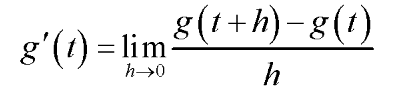Altogether what we're really doing is calculating the arc length of a curve as the sum of a bunch of hypotenuses of right triangles defined by tiny changes in x and y between points on the curve.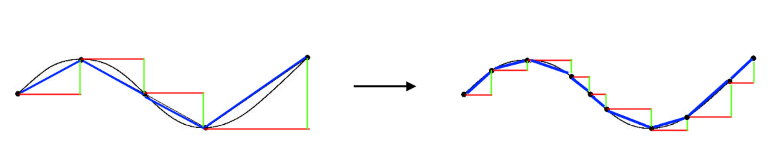Conclusion: So, since we care about length, maybe we should care about the how derivative of our curve at each point behaves as the series progresses... If the derivatives don't converge then length probably isn't going to converge.

Part 6 (THE LAST PART)

In the square-noose proof, by construction, the derivative of any differentiable point is either 0 or infinity. Therefore, the derivative function of the series does not convergence to match the circle's derivative function.

Additionally, the Spiral of Doom is a proof where both distance and the instantaneous derivatives of all of the points in the approximation series converge pointwise to the right values. This is because, in fact, the kind of convergence we need is uniform convergence, not just pointwise convergence.

The punch line:

As $n$ goes to $\infty,$ the length of an n-gon curve circumscribing a circle approaches the length of circle not just because all of the points on the n-gon are becoming arbitrarily close to points on the circle, nor even is it enough for all of the points to also be converging in slope to tangency with the circle. Instead, we actually need UNIFORM convergence of both the value of the series,$F_n(x,y)$ converging to the circle, $C(x,y),$ and of the derivative of the series function to the derivative of the circle: $F'_n(x,y)$ converging to $C'(x,y).$

I know, this distinction between pointwise and uniform convergence is super subtle but it's also how we prove pi doesn't equal 4 or anything other than $\pi$ in an unquestionable way. With definitions and precision in the steps of our proof that take us a level beyond what intuition is typically capable of!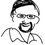Note by Jason Dyer
3 years, 2 months ago

This discussion board is a place to discuss our Daily Challenges and the math and science related to those challenges. Explanations are more than just a solution — they should explain the steps and thinking strategies that you used to obtain the solution. Comments should further the discussion of math and science.

When posting on Brilliant:

• Use the emojis to react to an explanation, whether you're congratulating a job well done , or just really confused .
• Ask specific questions about the challenge or the steps in somebody's explanation. Well-posed questions can add a lot to the discussion, but posting "I don't understand!" doesn't help anyone.
• Try to contribute something new to the discussion, whether it is an extension, generalization or other idea related to the challenge.
• Stay on topic — we're all here to learn more about math and science, not to hear about your favorite get-rich-quick scheme or current world events.

MarkdownAppears as
*italics* or _italics_ italics
**bold** or __bold__ bold
- bulleted- list
• bulleted
• list
1. numbered2. list
1. numbered
2. list
Note: you must add a full line of space before and after lists for them to show up correctly
paragraph 1paragraph 2

paragraph 1

paragraph 2

[example link](https://brilliant.org)example link
> This is a quote
This is a quote
    # I indented these lines
# 4 spaces, and now they show
# up as a code block.

print "hello world"
# I indented these lines
# 4 spaces, and now they show
# up as a code block.

print "hello world"
MathAppears as
Remember to wrap math in $$ ... $$ or $ ... $ to ensure proper formatting.
2 \times 3 $2 \times 3$
2^{34} $2^{34}$
a_{i-1} $a_{i-1}$
\frac{2}{3} $\frac{2}{3}$
\sqrt{2} $\sqrt{2}$
\sum_{i=1}^3 $\sum_{i=1}^3$
\sin \theta $\sin \theta$
\boxed{123} $\boxed{123}$

## Comments

There are no comments in this discussion.

×

Problem Loading...

Note Loading...

Set Loading...